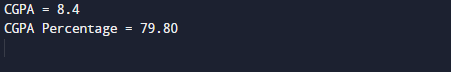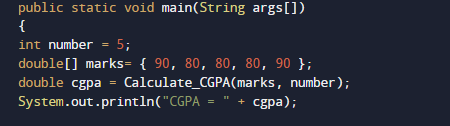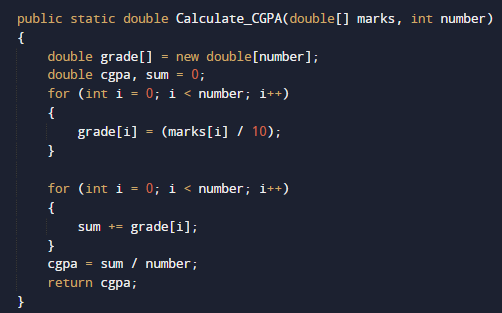# Java Program to Calculate CGPA

In this tutorial you will learn about the Java Program to Calculate CGPA and its application with practical example.

In this tutorial, we will learn to create a Java Program to Calculate CGPA using Java programming.

## Prerequisites

Before starting with this tutorial we assume that you are best aware of the following Java programming topics:

• Java Operators.
• Basic Input and Output function in Java.
• Class and Object in Java.
• Basic Java programming.
• If-else statements in Java.
• For loop in Java.

For conversion of CGPA to percentage, the CGPA must be multiplied with 9.5.

Example. if your score in Cgpa is 9.7 ,then in “%”, it will be 9.7×9.5=92.1%.

## Java Program to Calculate CGPA

In this program we will learn to create a program that will Calculate CGPA and Percentage from CGPA. Firstly we declare required variable and also initiates required values in variable. Next we take value from user at run time and then after we will find CGPA and Percentage from CGPA .Lets have a look at the program.

## OutputIn the above program, we have first declared and initialized a set of variables required in the program.

• number= it will hold size of number.
• marks= it will hold given marks.
• sum= it wil hold sum of values.
• cgpa= it will give value result in cgpa.

First of all we declared and initialized set of variables required in the program. Then we will take a value and pass this value to function Calculate_CGPA(marks,number).With in the Function we will Calculate both the answer in CGPA and also in percentage.And return the result back to main function where we print the value in CGPA and in Percentage.To get value form CGPA just simply multiply with 9.5 as shown in above picture.

In this tutorial we have learn about the Java Program to Calculate CGPA and its application with practical example. I hope you will like this tutorial.